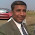## Monday, February 1, 2010

### Excel Formula : Sum top 5 in unsorted range

Today I am sharing a formula to sum top 5 values in an unsorted range.

=SUMPRODUCT(LARGE(Data,ROW(1:5)))

See the screen cast below to know secret behind this formula.Actually we are using array formula to find top 5 values , then summing them to find the sum of top 5 values in unsorted range.

From the screen cast you can see that

1. We are entering this formula using sumproduct, which is shortcut to enter array formulas in excel.
2. Data is a named range A3 to B36
3. We are using excel function Large to find Top values.
4. Row(1:5) - is is shortcut to create an array of numbers {1,2,3,4,5} as Kth position

This leads to find top 5 values in unsorted range and then summing them.

Food for thought :

Just change Row(1:5) to Row(1:10) you will be able to find Top 10 values.

Or Change Large with small to find out bottom 5 values.

### Download Excel file with formula to sum top 5 values in an unsorted range

to play furhter with this formula

1.we can use
=SUM(LARGE(Data;ROW(1:5)))
and click
Ctrl + Enter

2.sorry!
Ctrl +Shift+ Enter

3.Hello kemas

Thanks for your inpputs. I use sumproduct as workaround to enter CSE(Array) Formulas. The problem with the CSE formulas is exactly the same as you made mistake while writing this comment. In case user does not enter it with Ctrl+Shift+Enter it does not work.

Problem gets compounded when some one using your worksheet tries to modify your CSE formula. If that user is not aware of CSE formulas, he will never figure out why the formula stoped working.

Just write Sumproduct(Your CSE formula) all these issues disappear

Thanks

4.What if all the cells in your data range don't have data yet? is there a way to only total the ones that are there up to a certain number? I tried using something like =SUMPRODUCT(LARGE(E9:N9,ROW(INDIRECT("1:B2"))))
where B2 is a cell containing =COUNTIF(E9:N9,">0") to count how many data points exist at this point.

1.=SUMPRODUCT(LARGE(A2:A100,ROW(INDEX(A:A,1):INDEX(A:A,B2))))

SUMPRODUCT

...where B2 contains the number 3, indicating that you want to sum the top 3 numbers.

5.@ SmprFidels

Formula will not work the way you have constructed it. How ever if your data has less then 5 numbers you can use sum instead of sumproduct as above. This can be handled using if function.

I would have deployed following formula in situation like yours

=IF(COUNT(E9:N9)<5,SUM(E9:N9),(SUMPRODUCT(LARGE(E9:N9,ROW(1:5)))))

I hope this helps, let me know in case you need further help
Regards

6.I need to get top 3 positions in result sheet. I have a columns with Total Marks in which I sum all subjects marks Now I want to get top 3 positions from this column please guide me.

7.love this formula. thanks for sharing!

8.Thanks Yogesh. Found very helpful and learnt two new functions LARGE and ROW.

9.Hi

I have one small query in addition to this topic. If I have 4 values like

Marks obtained Total Marks
8 10
15 20
12 15
18 30

My question is:

First find the best three marks (as the total marks of each assignment (i,e out of marks like 10, 20, 15, 30) are different and obviously the % of each assignment would decide which are best three to choose)

Then those three marks have to sum together (based on the three largest % marks) and then get the final % of the sum out of total marks (corresponding)?

BACKGROUND:

As students gave me the assignments 4 and all assignments have different total marks (i,e out of marks like 10, 20, 15, 30). Now I want first to select the best three assignment marks (which is just possible by calculating their % of each as total marks are different). Then sum those three marks and then get the % of them by using the corresponding total marks of each of them.

10.